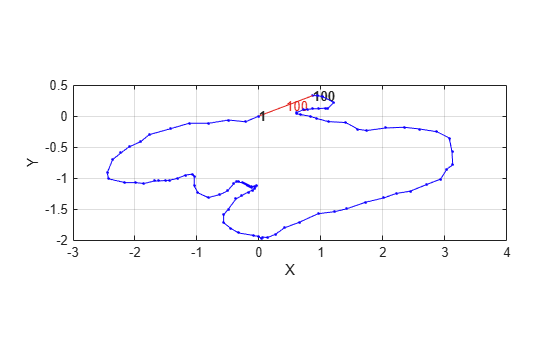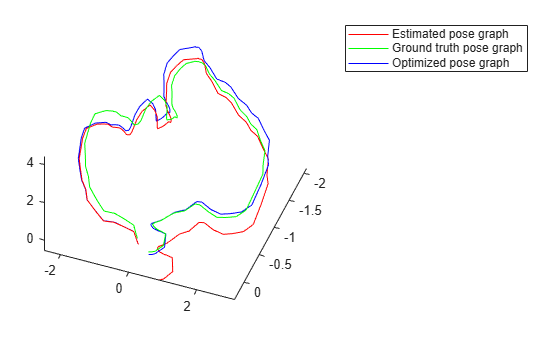Documentation

## Reduce Drift in 3-D Visual Odometry Trajectory Using Pose Graphs

This example shows how to reduce the drift in the estimated trajectory (location and orientation) of a monocular camera using 3-D pose graph optimization. Visual odometry estimates the current global pose of the camera (current frame). Because of poor matching or errors in 3-D point triangulation, robot trajectories often tends to drift from the ground truth. Loop closure detection and pose graph optimization reduce this drift and correct for errors.

### Load Estimated Poses for Pose Graph Optimization

Load the estimated camera poses and loop closure edges. Estimated camera poses are computed using visual odometry. Loop closure edges are computed by finding previous frame which saw the current scene and estimating the relative pose between the current frame and the loop closure candidate. Camera frames are sampled from .

```% Estimated poses load('estimatedpose.mat'); % Loopclosure edge load('loopedge.mat'); % Groundtruth camera locations load('groundtruthlocations.mat');```

### Build 3-D Pose Graph

Create an empty pose graph.

`pg3D = poseGraph3D;`

Add nodes to the pose graph, with edges defining the relative pose and information matrix for the pose graph. Convert the estimated poses, given as transformations, to relative poses as an `[x y theta qw qx qy qz]` vector. An identity matrix is used for the information matrix for each pose.

```len = size(estimatedPose,2); informationmatrix = [1 0 0 0 0 0 1 0 0 0 0 1 0 0 0 1 0 0 1 0 1]; % Insert relative poses between all successive frames for k = 2:len % Relative pose between current and previous frame relativePose = estimatedPose{k-1}/estimatedPose{k}; % Relative orientation represented in quaternions relativeQuat = tform2quat(relativePose); % Relative pose as [x y theta qw qx qy qz] relativePose = [tform2trvec(relativePose),relativeQuat]; % Add pose to pose graph addRelativePose(pg3D,relativePose,informationmatrix); end```

Add a loop closure edge. Add this edge between two existing nodes from the current frame to a previous frame.

```% Convert pose from transformation to pose vector. relativeQuat = tform2quat(loopedge); relativePose = [tform2trvec(loopedge),relativeQuat]; % Loop candidate loopcandidateframeid = 1; % Current frame currentframeid = 100; addRelativePose(pg3D,relativePose,informationmatrix,... loopcandidateframeid,currentframeid); figure show(pg3D);```Optimize the pose graph. The nodes are adjusted based on the edge constraints to improve the overall pose graph. To see the change in drift, plot the estimated poses and the new optimized poses against the ground truth.

```% Pose graph optimization optimizedPosegraph = optimizePoseGraph(pg3D); optimizedposes = nodes(optimizedPosegraph); % Camera trajectory plots figure estimatedposes = nodes(pg3D); plot3(estimatedposes(:,1),estimatedposes(:,2),estimatedposes(:,3),'r'); hold on plot3(groundtruthlocations(:,1),groundtruthlocations(:,2),groundtruthlocations(:,3),'g'); plot3(optimizedposes(:,1),optimizedposes(:,2),optimizedposes(:,3),'b'); hold off legend('Estimated pose graph','Ground truth pose graph', 'Optimized pose graph'); view(-20.8,-56.4);```### References

 Galvez-López, D., and J. D. Tardós. "Bags of Binary Words for Fast Place Recognition in Image Sequences." IEEE Transactions on Robotics. Vol. 28, No. 5, 2012, pp. 1188-1197.